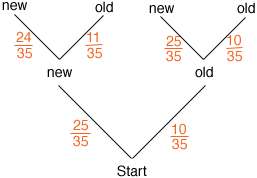Quandaries and Queries Ive been having some trouble figuring this one out. I have a hopper of tennis balls that contains 25 new tennis balls and 10 old tennis balls. In the hopper the tennis balls are mixed up randomly. If i take one ball out of the hopper and hit it and then put it back and then take another ball out of the hopper and hit it and put it back, what is the probability that i hit two new tennis balls? Also a tennis ball becomes old after being hit just once. Hi Jeremy, I would draw a tree diagram to describe this two step process.You start with 35 balls, 25 new and 10 old. At the first level of the tree you choose one ball at random. The probability that it is a new ball is 25/35 and the probability that it is an old ball is 10/35. The probabilities at the second level depend on whether you chose a new or old ball at the first level. If you chose an old ball at the first level, hit it and the put it back, the situation is unchanged. You still have 25 new balls and 10 old balls so the probabilities at the second level are the same as they were at the first level. If you chose a new ball at the first level, hit it and put it back, you have changed that ball to an old ball. In this case the balls to chose from at the second level are 11 old and 24 new. Thus the probability of chosing a new ball at the second level, if you chose a new ball at the first level, is 24/35. Hence the probability of chosing a new ball on the first level and a new ball on the second level is  25/3524/35 = (2524)/352 Penny Go to Math Central